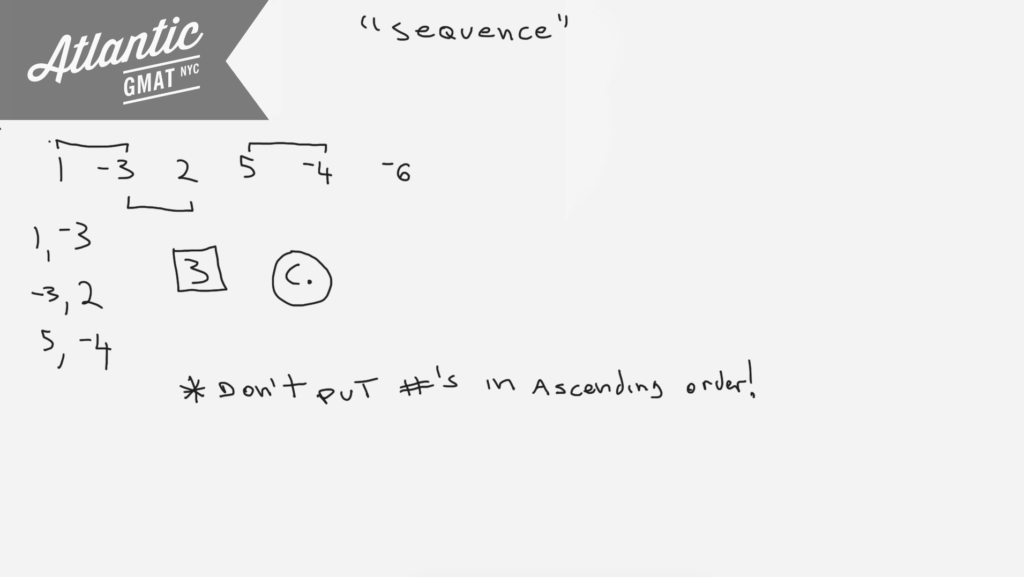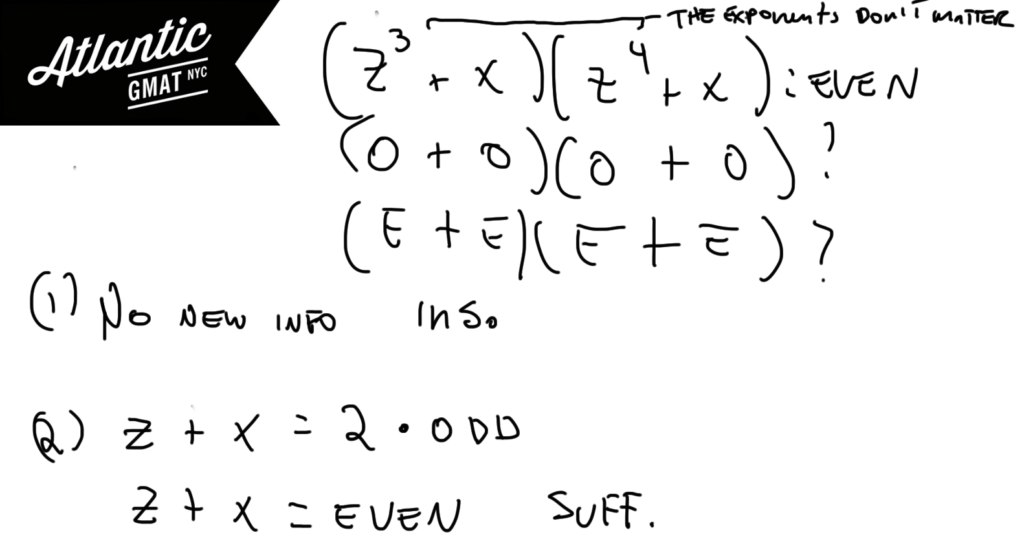Private GMAT Tutoring in NYC & Online

For a finite sequence of non zero numbers, the number of variations in sign is defined as the number of pairs of consecutive terms of the sequence for which the product of the two consecutive terms is negative. What is the number of variations in sign for the sequence 1, -3, 2, 5, -4, -6 ?

(A) 1
(B) 2
(C) 3
(D) 4
(E) 5The number 75 can be written as the sum of the squares of 3 different positive integers. What is the sum of these 3 integers?

(A) 17
(B) 16
(C) 15
(D) 14
(E) 13

This question is from the free official GMAT practice tests 1 and 2 so avoid if you haven't done those already. This is a number properties puzzle that benefits from doing a bit of organizing. The sum of the squares of the 3 integers sum is 75. So go ahead and list the first bunch of squares starting from 1 up to just below 75. Once you've got that you just need to find the combination that works. To make your job easier use units digits. 75 ends in 5 so your three numbers must create that units digit. The other tip is: start from the bigger integers and work down. To get to 75 you're going to need one of those big guys (64, 49, or 36). Finding a constraint can be very helpful (in this case the units digit and the requirement of 36, 49, or 64). Usually with these GMAT puzzles there are little shortcuts. Generally you don't pull them out of thin air. They are the natural conclusion of good organization. Make sure to take your time with setup so you pave the way for success. Here's another GMAT question that relies on just staying organized and paying attention: For a certain race, 3 teams were allowed to enter 3 members each. A team earned 6 – n points whenever one of its members finished in nth place, where 1 ≤ n ≤ 5. The details are very different but the style is similar and I think it requires the same kind of thinking.# GMAT Question of the Day - Data Sufficiency - Divisibility

What is the greatest common factor of positive integers k and m?

(1) When integer k is divided by two the result is an integer with no prime factors.

(2) m = 25

# GMAT Question of the Day - Data Sufficiency - Number Properties

If z and x are integers is (z^3 + x)(z^4 + x) even?

(1) x^3 + x^2 is even

(2) (z^2 + x^2)/2 is odd

## GMAT Question of the Day Solution

Simplify GMAT DS questions. Break down what the question is asking. Take this seriously. It's your job on DS questions to figure out how to simplify the question. On this GMAT question of the day we're really being asked if z + x is even which further breaks down to: Do x and z have the same parity? What about the exponents? Provided that the numbers themselves are integers and that the exponents are positive integers the exponents do not change the oddness or evenness. Usually the GMAT puts in exponents to distract you from the real question.

Statement (1) only tells about x. Insufficient. Statement (2) tells us that z + x must be even. Why? When divided by 2 only an even number can result in an integer. In this case that even number only has one two as a factor and so when divided by two the result is an odd number. So the given expression must be even. The answer is yes. Sufficient.# GMAT Question of the Day - Data Sufficiency - Number Properties

If x is a positive integer greater than 1 does x have more than two factors?

(1) x has the same number of factors as y^2

(2) The result when y is divided by x is an integer

# CONTACT

Atlantic GMAT

405 East 51st St.

NY, NY 10022

(347) 669-3545

info@AtlanticGMAT.com